# Mann Whitney Testing with JMP

### What is Mann Whitney Testing with JMP?

Mann Whitney testing with JMP (also called Mann–Whitney U test or Wilcoxon rank-sum test) is a statistical hypothesis test to compare the medians of two populations that are not normally distributed. In a non-normal distribution, the median is the better representation of the center of the distribution.

• Null Hypothesis (H0): η1 = η2
• Alternative Hypothesis (Ha): η1η2

Where:

•  η1 is the median of one population
• η2 is the median of the other population
• The null hypothesis is that the medians are equal, and the alternative is that they are not equal.

### Assumptions for Mann Whitney Testing with JMP

• The sample data drawn from the populations of interest are unbiased and representative
• The data of both populations are continuous or ordinal when the spacing between adjacent values is not constant (Reminder: Ordinal data—A set of data is said to be ordinal if the values can be ranked or have a rating scale attached. You can count and order, but not measure ordinal data)
• The two populations are independent of each other
• The Mann–Whitney test is robust for the non-normally distributed population.
• The Mann–Whitney test can be used when the shapes of the two populations’ distributions are different.

### How Mann Whitney Test Works

Step 1:
Group the two samples from two populations (sample 1 is from population 1 and sample 2 is from population 2) into a single data set. Then, sort the data in ascending order ranked from 1 to n, where n is the total number of observations.
Step 2:
Add up the ranks for all the observations from sample 1 and call it R1. Add up the ranks for all the observations from sample 2 and call it R2 .
Step 3:
Calculate the test statistics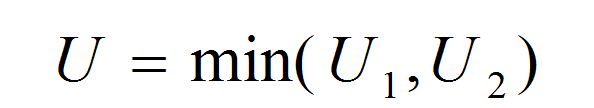Where: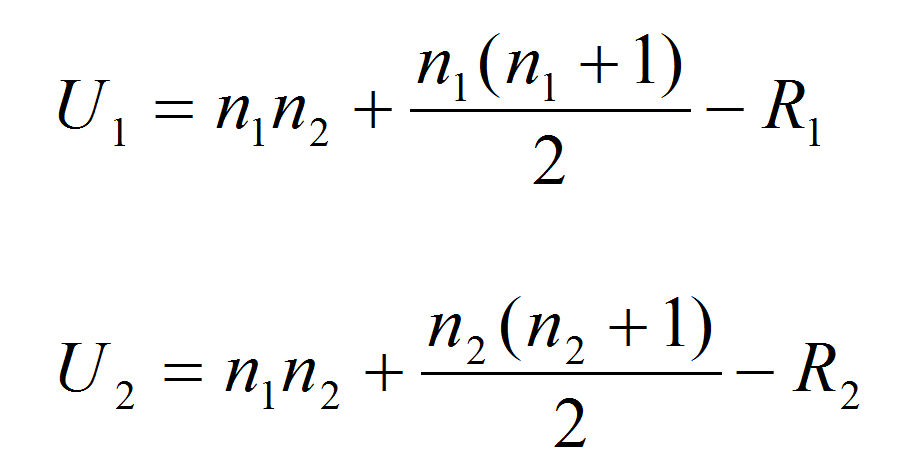and where:

• η1 and η2 are the sample sizes
• R1 and R2 are the sum of ranks for observations from samples 1 and 2, respectively

Step 4:
Decide on whether to reject the null hypothesis

• Null Hypothesis (H0): η1 = η2
• Alternative Hypothesis (Ha): η1η2

If both sample sizes are smaller than 10, the distribution of U under the null hypothesis is tabulated.

• The test statistic is U, and by using the Mann–Whitney table, we would find the p-value.
• If the p-value is smaller than the alpha level (0.05), we reject the null hypothesis.
• If the p-value is greater than the alpha level (0.05), we fail to reject the null hypothesis
• If both sample sizes are greater than 10, the distribution of U can be approximated by a normal distribution. In other words, (U-μ)/σ follows a standard normal distribution.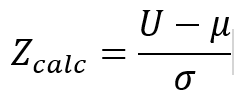Where: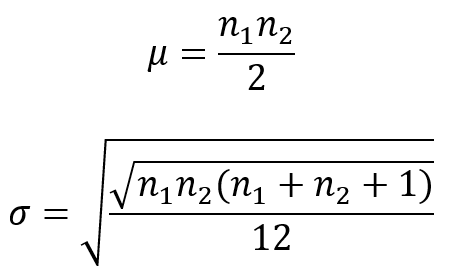If the sample sizes are greater than 10, then the distribution of U can be approximated by a normal distribution. The U value is then plugged into the formula here to calculate a Z statistic.
When |Zcalc| is greater than the Z value at α/2 level (e.g., when α = 5%, the z value we compare |Zcalc| to is 1.96), we reject the null hypothesis.

### Mann–Whitney Testing with JMP

Case study: We are interested in comparing customer satisfaction between two types of customers using a nonparametric (i.e., distribution-free) hypothesis test: Mann–Whitney test.
Data File: "Mann–Whitney.jmp”Fig 1.0 Mann-Whitney Test

• Null Hypothesis (H0): η1 = η2
• Alternative Hypothesis (Ha): η1η2

#### Steps to run a Mann–Whitney Test in JMP:

1. Click Analyze -> Fit Y by X
2. Select “Overall Satisfaction” as “Y, Response”
3. Select “Customer Type” as “X, Factor”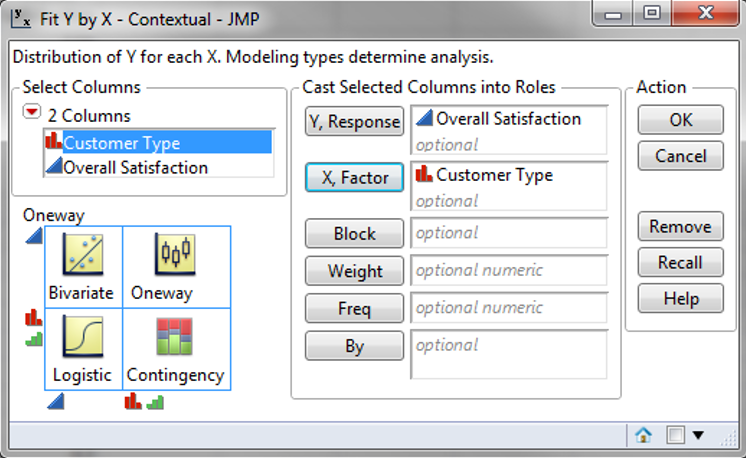4. Click “OK”
5. Click on the red triangle button next to “One-Way Analysis of Overall Satisfaction by Customer Type”
6. Click Nonparametric -> Wilcoxon Test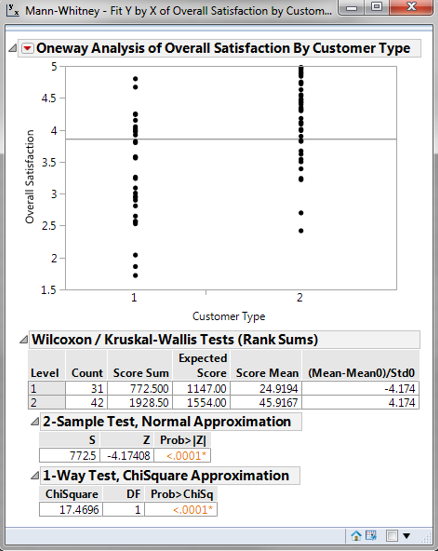Model summary: The p-value of the test is lower than the alpha level (0.05), so we reject the null hypothesis and conclude that there is a statistically significant difference between the overall satisfaction medians of the two customer types.

The result of the test is boxed in. The p-value is lower than the alpha value of 0.05; therefore, we must reject the null hypothesis and claim that there is a significant difference between the median customer satisfaction levels of the two groups.